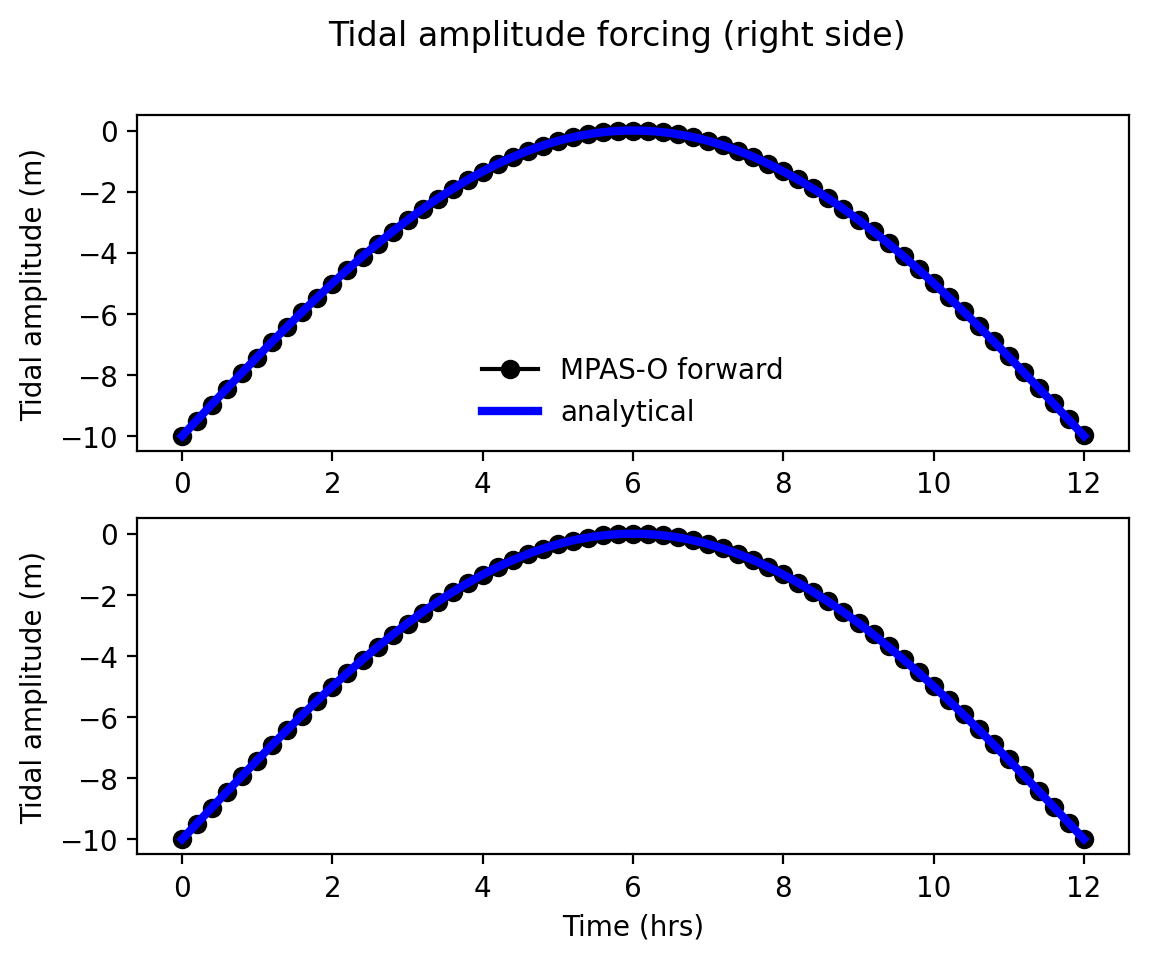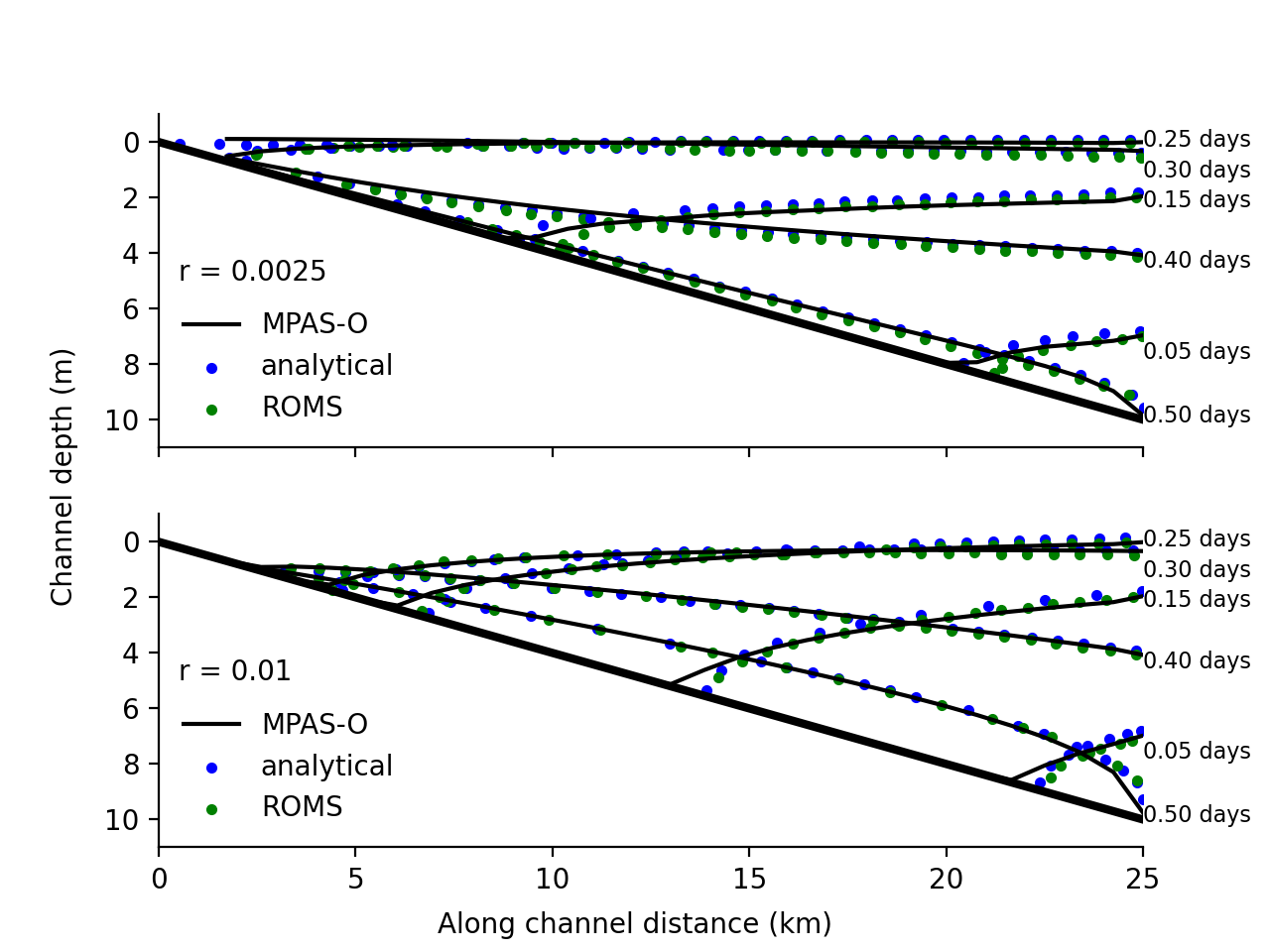# drying_slope¶

The ocean/drying_slope test group reproducs the tidal channel flow test case from Warner et al. (2013) which was originally presented in Oey (2005).

The domain is 2-d with a channel sloping in the x-dimension and periodic in the y-dimension. The channel length is set to 25 km and slopes with an aspect ratio of 0.1. The channel width is 6km for all simulations. The channel is initialized as dry with a minimum layer thickness. The water level at the seaward end is oscillated sinusoidally with a period of 0.5 days as

$\zeta_{east} = 10.0 \sin(\pi/0.5t) - 10.0$Temperature and salinity are constant throughout the domain with values of 20 degC and 35 PSU, respectively. Two different linear drag bottom roughness factors of 0.0025 and 0.01 are applied in separate simulations.

By default, there are 10 vertical layers. The sigma vertical coordinate is used. Horizontal resolution of 250m and 1 km are available.

The test group includes 1 test case. The test case has 4 steps. The initial_state defines the mesh and initial conditions for the model. For the sigma vertical coordiante, two forward steps are run, one for each Rayleigh damping coefficient; for the single_layer case, one forward step is run at one value of the explicit bottom drag coefficient. The viz step performs visualization of the ssh field. For the sigma case, the viz step also produces a comparison plot with the analytical solution as well as the solution from the ROMS model.## config options¶

The config options for this test case are:

# Options related to the vertical grid
[vertical_grid]

# Number of vertical levels
vert_levels = 10

# The type of vertical coordinate (e.g. z-level, z-star)
coord_type = sigma

# config options for drying slope test cases
[drying_slope]

# the number of grid cells in x and y
nx = 6

# config options for visualizing drying slope ouptut
[drying_slope_viz]

# whether to generate movie
generate_movie = False

# frames per second for movies
frames_per_second = 30

# movie format
movie_format = mp4


All units are mks.

## default¶

ocean/drying_slope/${RES}/${COORD}/default is the default version of the drying slope test case for two short (12h) test runs with two different drag coefficients and validation of sea surface height through visual inspection against analytic and ROMS solutions. RES is either 250m or 1km. COORD is either single_layer or sigma. Rayleigh drag is not compatible with single_layer so implicit drag with a constant coefficient is used.

## ramp¶

ocean/drying_slope/${RES}/${COORD}/ramp is identical to the default test except the factor that scales velocities and velocity tendencies is ramped over a given layer thickness range rather than a binary switch at the minimum thickness. RES is either 250m or 1km. COORD is either single_layer or sigma.

## loglaw¶

ocean/drying_slope/${RES}/${COORD}/loglaw is identical to the default test except that the log-law option for implicit bottom drag is used. RES is either 250m or 1km. COORD is either single_layer or sigma.